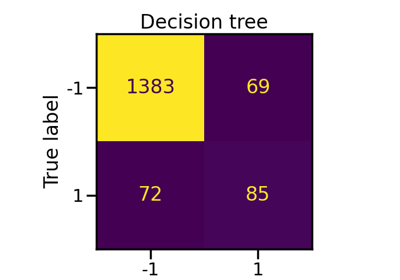# imblearn.ensemble.RUSBoostClassifier¶

class imblearn.ensemble.RUSBoostClassifier(base_estimator=None, *, n_estimators=50, learning_rate=1.0, algorithm='SAMME.R', sampling_strategy='auto', replacement=False, random_state=None)[source]

Random under-sampling integrated in the learning of AdaBoost.

During learning, the problem of class balancing is alleviated by random under-sampling the sample at each iteration of the boosting algorithm.

Read more in the User Guide.

Parameters
base_estimatorobject, default=None

The base estimator from which the boosted ensemble is built. Support for sample weighting is required, as well as proper classes_ and n_classes_ attributes. If None, then the base estimator is DecisionTreeClassifier(max_depth=1).

n_estimatorsint, default=50

The maximum number of estimators at which boosting is terminated. In case of perfect fit, the learning procedure is stopped early.

learning_ratefloat, default=1.0

Learning rate shrinks the contribution of each classifier by learning_rate. There is a trade-off between learning_rate and n_estimators.

algorithm{‘SAMME’, ‘SAMME.R’}, default=’SAMME.R’

If ‘SAMME.R’ then use the SAMME.R real boosting algorithm. base_estimator must support calculation of class probabilities. If ‘SAMME’ then use the SAMME discrete boosting algorithm. The SAMME.R algorithm typically converges faster than SAMME, achieving a lower test error with fewer boosting iterations.

sampling_strategyfloat, str, dict, callable, default=’auto’

Sampling information to sample the data set.

• When float, it corresponds to the desired ratio of the number of samples in the minority class over the number of samples in the majority class after resampling. Therefore, the ratio is expressed aswhereis the number of samples in the minority class andis the number of samples in the majority class after resampling.

Warning

float is only available for binary classification. An error is raised for multi-class classification.

• When str, specify the class targeted by the resampling. The number of samples in the different classes will be equalized. Possible choices are:

'majority': resample only the majority class;

'not minority': resample all classes but the minority class;

'not majority': resample all classes but the majority class;

'all': resample all classes;

'auto': equivalent to 'not minority'.

• When dict, the keys correspond to the targeted classes. The values correspond to the desired number of samples for each targeted class.

• When callable, function taking y and returns a dict. The keys correspond to the targeted classes. The values correspond to the desired number of samples for each class.

replacementbool, default=False

Whether or not to sample randomly with replacement or not.

random_stateint, RandomState instance, default=None

Control the randomization of the algorithm.

• If int, random_state is the seed used by the random number generator;

• If RandomState instance, random_state is the random number generator;

• If None, the random number generator is the RandomState instance used by np.random.

BalancedBaggingClassifier

Bagging classifier for which each base estimator is trained on a balanced bootstrap.

BalancedRandomForestClassifier

Random forest applying random-under sampling to balance the different bootstraps.

EasyEnsembleClassifier

Ensemble of AdaBoost classifier trained on balanced bootstraps.

References

1

Seiffert, C., Khoshgoftaar, T. M., Van Hulse, J., & Napolitano, A. “RUSBoost: A hybrid approach to alleviating class imbalance.” IEEE Transactions on Systems, Man, and Cybernetics-Part A: Systems and Humans 40.1 (2010): 185-197.

Examples

>>> from imblearn.ensemble import RUSBoostClassifier
>>> from sklearn.datasets import make_classification
>>>
>>> X, y = make_classification(n_samples=1000, n_classes=3,
...                            n_informative=4, weights=[0.2, 0.3, 0.5],
...                            random_state=0)
>>> clf = RUSBoostClassifier(random_state=0)
>>> clf.fit(X, y)
RUSBoostClassifier(...)
>>> clf.predict(X)
array([...])

Attributes
base_estimator_estimator

The base estimator from which the ensemble is grown.

estimators_list of classifiers

The collection of fitted sub-estimators.

samplers_list of RandomUnderSampler

The collection of fitted samplers.

pipelines_list of Pipeline

The collection of fitted pipelines (samplers + trees).

classes_ndarray of shape (n_classes,)

The classes labels.

n_classes_int

The number of classes.

estimator_weights_ndarray of shape (n_estimator,)

Weights for each estimator in the boosted ensemble.

estimator_errors_ndarray of shape (n_estimator,)

Classification error for each estimator in the boosted ensemble.

feature_importances_ndarray of shape (n_features,)

The impurity-based feature importances.

__init__(self, base_estimator=None, *, n_estimators=50, learning_rate=1.0, algorithm='SAMME.R', sampling_strategy='auto', replacement=False, random_state=None)[source]

Initialize self. See help(type(self)) for accurate signature.

## Examples using imblearn.ensemble.RUSBoostClassifier¶